# angle

(redirected from distal a)
Also found in: Dictionary, Thesaurus, Legal, Encyclopedia.
Related to distal a: distal myopathy

## angle

[ang´g'l]
the space or figure formed by two diverging lines, measured as the number of degrees one would have to be moved to coincide with the other.
acromial angle that between the head of the humerus and the clavicle.
alpha angle that formed by intersection of the visual axis with the optic axis.
buccal a's
1. the tooth angles between the buccal surface and the other surfaces of a posterior tooth; see accompanying illustration.
2. the cavity angles between the buccal wall of a tooth cavity and other walls.
cardiodiaphragmatic angle that formed by the junction of the shadows of the heart and diaphragm in posteroanterior radiographs of the heart.
cavity a's the angles formed by the junction of two or more walls of a tooth cavity, named according to the walls participating in their formation.
cavosurface angle the angle formed by the junction of a wall of a tooth cavity preparation and a surface of the crown of the tooth.
costovertebral angle the angle formed on either side of the vertebral column between the last rib and the lumbar vertebrae.
distal a's
1. the tooth angles formed between the distal surface and the other surfaces of a tooth; see accompanying illustration.
2. the cavity angles between the distal wall of a tooth cavity and other walls.
filtration angle (angle of the iris) the angle between the iris and cornea at the periphery of the anterior chamber of the eye, through which the aqueous humor readily permeates.
angle of jaw the junction of the lower edge with the posterior edge of the lower jaw.
lingual a's
1. the tooth angles formed between the lingual surface and the other surfaces of a tooth; see accompanying illustration.
2. the cavity angles between the lingual wall of a tooth cavity preparation and other walls.
angle of Louis an anatomical landmark located on the sternum; it can be felt as a notch or ridge at the top of the sternum.
mesial a's
1. the tooth angles formed between the mesial surface and other surfaces of a tooth; see accompanying illustration.
2. the cavity angles between the mesial wall of a tooth cavity and other walls.
meter angle the angle formed by intersection of the visual axis and the perpendicular bisector of the line joining the centers of rotation of the two eyes when viewing a point one meter distant (small meter angle) or the angle formed by intersection of the visual axes of the two eyes in the midline at a distance of one meter (large meter angle).
optic angle visual angle.
angle of pubis that between the pubic bones at the symphysis.
sternoclavicular angle that between the sternum and the clavicle.
tooth a's the angles formed by the junction of two or more surfaces of a tooth, named according to the surfaces participating in their formation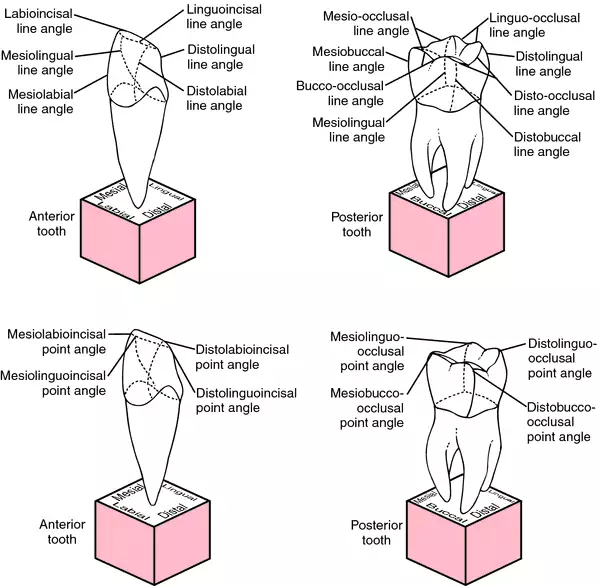Tooth angles: Top, line angles; Bottom, point angles. From Dorland's, 2000.
(see accompanying illustration).
visual angle the angle between two lines passing from the edges of an object seen, through the nodal point of the eye, to the corresponding edges of the image of the object seen.

## An·gle

(ang'gĕl),
Edward Hartley, U.S. orthodontist, 1855-1930. See: Angle classification of malocclusion.

## an·gle (θ),

(ang'gl), [TA]
The meeting point of two lines or planes; the figure formed by the junction of two lines or planes; the space bounded on two sides by converging lines or planes. For angles not listed below, see the descriptive term; for example, axioincisal, distobuccal, labiogingival, linguogingival (2), mesiogingival, and proximobuccal.
Synonym(s): angulus [TA]
[L. angulus]

## angle

/an·gle/ (ang´g'l)
1. the point at which two intersecting borders or surfaces converge.
2. the degree of divergence of two intersecting lines or planes.

acromial angle  the subcutaneous bony point at which the lateral border becomes continuous with the spine of the scapula.
axial angle  any line angle parallel with the long axis of a tooth.
cardiodiaphragmatic angle  that formed by the junction of the shadows of the heart and diaphragm in posteroanterior radiographs of the chest.
costovertebral angle  that formed on either side of the vertebral column between the last rib and the lumbar vertebrae.
angle of eye  canthus.
filtration angle  a narrow recess between the sclerocorneal junction and the attached margin of the iris, at the periphery of the anterior chamber of the eye; it is the principal exit site for the aqueous fluid.
iridial angle , iridocorneal angle, angle of iris filtration a.
line angle  an angle formed by the junction of two planes; in dentistry, the junction of two surfaces of a tooth or of two walls of a tooth cavity.
Louis' angle , Ludwig's angle sternal a.
optic angle  visual a.
point angle  one formed by the junction of three planes; in dentistry, the junction of three surfaces of a tooth, or of three walls of a tooth cavity.
angle of pubis  subpubic a.
sternal angle  the angle between the sternum and manubrium.
subpubic angle  that formed by the conjoined rami of the ischial and pubic bones.
tooth angles  those formed by two or more tooth surfaces.
venous angle  the angle formed by junction of the internal jugular and subclavian veins.
visual angle  the angle formed between two lines extending from the nodal point of the eye to the extremities of the object seen.
Y angle  that between the radius fixus and the line joining the lambda and inion.

## angle

[ang′gəl]
Etymology: L, angulus
1 the space or the shape formed at the intersection of two lines, planes, or borders. The divergence of the lines, planes, or borders may be measured in degrees of a circle.
2 (in anatomy and physiology) the geometric relationships between the surfaces of body structures and the positions affected by movement.

## an·gle

(ang'gĕl) [TA]
The figure formed by the junction of two lines or planes; the space bounded on two sides by lines or planes that meet. For specific angles, see the descriptive term, e.g., axioincisal, distobuccal, labiogingival, linguogingival (2), mesiogingival, proximobuccal.
Synonym(s): angulus [TA] .
[L. angulus]

## angle

(ang'gel) [L. angulus, corner, angle]
1. The figure or space outlined by the diverging of two lines from a common point or by the meeting of two planes.
2. A projecting or sharp corner.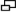A ANGLE: The A angle is the relationship between the long axis of the patella and the tibial tuberosity; the Q angle describes the relationship between the long axis of the femur, measured from the anterior superior ilac spine.

### A angle

The orientation of the patella relative to the tibial tubercle. The angle is formed by the intersection of a line bisecting the long axis of the patella and a passed through the tibial tubercle to the apex of the inferior pole of the patella.
See: illustration

### acromial angle

The angle formed by the junction of the lateral and posterior borders of the acromion.

### acute angle

An angle less than 90°.

### alpha angle

The angle formed by intersection of the visual line with the optic axis.

### alveolar angle

The angle between the horizontal plane and a line drawn through the base of the nasal spine and the middle point of the alveolus of the upper jaw.

### anterior chamber angle

The angle between the cornea and iris at the periphery of the anterior chamber of the eye.

### biorbital angle

The angle formed by the meeting of the axes of the orbits.

### cardiophrenic angle

The medial inferior corner of the pulmonary cavity bordered by the heart and diaphragm.

### carrying angle

The angle in the sagittal plane made at the elbow by extending the long axis of the forearm and the upper arm. This obtuse angle is more pronounced in women than in men. Synonym: carrying angle of arm.

### caudal angle

In radiology, angulation of the central ray toward the patient's feet.

### cavity angle

The angle formed by two or more walls of a cavity preparation in restorative dentistry.

### cephalometric angle

The angle formed by intersecting anthropometric lines. It is used in studies of the skull and for the diagnosis of malocclusions of dental, skeletal, and dentoskeletal origin.

### cerebellopontine angle

The angle formed by the junction of the cerebellum and the pons. Synonym: pontine angle

### cervicofemoral angle

See: angle of inclination

See: Cobb angle

### angle of convergence

The angle between the visual axis and the median line when an object is looked at.

### costal angle

The meeting point of the lower border of the false ribs with the axis of the sternum.

### costophrenic angle

The lateral inferior corner of the pulmonary cavity bordered by the ribs and diaphragm.

### costovertebral angle

The angle formed on each side of the trunk by the junction of the last rib with the lumbar vertebrae.

### craniofacial angle

The angle formed by the basifacial and basicranial axes at the midpoint of the sphenoethmoidal suture.

### facial angle

The angle made by lines from the nasal spine and external auditory meatus meeting between the upper middle incisor teeth.

### flat angle

The angle between two lines that join at an angle of almost 180°.

### gamma angle

The angle between the line of vision and the optic axis.

Angle of jaw.

### Hilgenreiner epiphyseal angle

See: Hilgenreiner epiphyseal angle

### angle of incidence

The angle between a ray striking a surface and a line drawn perpendicular to the surface at the point of incidence.

### angle of inclinication (of the hip)

The angle between the femoral neck and the shaft of the femur. This angle is normally 35° in infancy; with maturation of the skeleton it increases to 45°.
Synonym: cervicofemoral angle

### angle of jaw

The angle formed where the vertical back edge of the ramus of the mandible meets the horizontal edge along the bottom. Synonym: gonial angle; angle of mandible

Angle of jaw.

### metafacial angle

The angle between the base of the skull and the pterygoid process.

### obtuse angle

An angle greater than 90°.

### occipital angle

The angle formed at the opisthion by the intersection of lines from the basion and from the lower border of the orbit.

### ophryospinal angle

The angle formed at the anterior nasal spine by the intersection of lines drawn from the auricular point and the glabella.

### parietal angle

The angle formed by the meeting of a line drawn tangent to the maximum curve of the zygomatic arch and a line drawn tangent to the end of the maximum frontal diameter of the skull. If these lines are parallel, the angle is zero; if they diverge, a negative angle is formed.

### pontine angle

Cerebellopontine angle.

### prophy angle

In dentistry, a wheel containing pieces of wire. It is used for cleaning metal surfaces.

### pubic angle

The angle formed by the junction of the rami of the pubic bones.

### Q angle

The acute angle formed by a line from the anterior superior iliac spine of the pelvis through the center of the patella and a line from the tibial tubercle through the patella. The angle describes the tracking of the patella in the trochlear groove of the femur. In women, the Q angle should be less than 22° with the knee in extension and less than 9° with the knee in 90° of flexion. In men, the Q angle should be less than 18° with the knee in extension and less than 8° with the knee in 90° of flexion. See: A angle for illus

### angle of refraction

The angle formed by a refracted ray of light with a line perpendicular to the surface at the refraction point.

An angle of 90°.

### sacrolumbar angle

The angle formed by articulation of the last lumbar vertebra and the sacrum.

### sacrovertebral angle

The angle formed by the base of the sacrum and the fifth lumbar vertebra.

### sphenoid angle

The angle formed at the top of the sella turcica by the intersection of lines drawn from the nasal point and the tip of the rostrum of the sphenoid.

### sternal angle

The angle formed by the junction of the manubrium and the body of the sternum.

### angle of Treitz

The sharp curve at the duodenojejunal junction.

### venous angle

The angle formed by the junction of the internal jugular and subclavian veins.

### visual angle

The angle between the line of sight and the extremities of the object seen.

## angle,

n the divergence of two lines or planes from a common point of origin.
angle of the sacrum, inferior lateral,
n the point on the lateral surface of the sacrum as it curves toward the middle of the fifth sacral vertebra.

## angle

1. The figure formed by two intersecting lines or planes at one point.
2. The direction from which an object is viewed.
3. The form produced by a change in direction of a line or plane.
angle alpha Angle between the visual axis and the optical axis formed at the first nodal point of the eye. The visual axis usually lies nasal to the optical axis on the plane of the cornea (positive angle alpha). It is, on average, equal to about 5º in the adult eye. If it lies temporal to the optical axis, the angle is denoted negative (Fig. A9). Symbol: α. See angle lambda.
angle of altitude 1. The angle through which the eyes have turned up or down from the primary position by a rotation about the transverse axis (x-axis). 2. The angle between the plane of regard and the subjective horizontal plane. Syn. angle of elevation.
angle of anomaly Angle between the line of visual direction of the fovea and the line of visual direction of the abnormal corresponding point of the same deviated eye. It is usually represented by the difference between the objective and subjective angles of deviation in abnormal retinal correspondence. See line of direction; abnormal retinal correspondence.
angle of the anterior chamber Angle at the periphery of the anterior chamber formed by the root of the iris, the front surface of the ciliary body and the trabecular meshwork. Syn. angle of filtration; drainage angle; irido-corneal angle. See gonioscope; Shaffer and Schwartz van Herick method; Smith's method; shadow test.
apical angle See prism angle.
angle of azimuth The angle through which the eyes have turned right or left from the primary position by a rotation about the vertical axis.
Brewster's angle See angle of polarization.
contact angle Angle formed by a surface and a tangent to a sessile drop of fluid (usually water) at the point where the drop meets the surface. This angle indicates the degree of wettability of that surface. The more wettable (or hydrophilic) the material, the smaller the angle, being equal to 0º for a completely hydrophilic material when water spreads evenly over that surface. Hydrophobic surfaces can have contact angles greater than 90º, e.g. silicone rubber in which the angle is about 120º (Fig. A10). Syn. wetting angle. See sessile drop test.
angle of convergence Angle between the lines of sight of the two eyes which are in a state of convergence. The angle is positive when the lines of sight intersect in front of the eyes, and negative when they intersect behind the eyes. Note: some authors regard the angle of convergence as the rotation of one eye only towards the fixation point, and refer to the angle of convergence of both eyes, defined above, as the total angle of convergence or the total convergence. The total angle of convergence required for binocular fixation of a target is equal to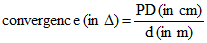or
convergence (in Χ) = convergence (in ma)✕ PD (in cm)
where d is the distance between the target and the midpoint of the base line and PD the interpupillary distance. Syn. angle of triangulation. See metre angle; prism dioptre; line of sight.
critical angle That angle of incidence which results in the refracted ray travelling along the surface between the two media (angle of refraction equal to 90º). If the angle of incidence is greater than the critical angle, the ray is totally reflected. If, however, the angle of incidence is smaller than the critical angle, the ray is refracted (with some light reflected). The critical angle ic is given by the following formula
sin ic = n/n
where n and n' are the indices of the media on each side of the surface, with the light travelling from the high index medium n to the low index medium n′ (Fig. A11). Syn. limiting angle. See fibre optics; reflecting prism; total reflection; refractometer.
angle of deviation 1. Angle through which a ray of light is deviated on reflection by a mirror, or refraction by a lens or prism. 2. Angle between the visual axis (or line of sight) of the deviated eye in strabismus and the straight-ahead position while the other eye fixates straight ahead. It can be assessed subjectively by having the patient report simultaneous perception (e.g. the lion in the cage seen in the amblyoscope) or objectively as measured by the practitioner either with the amblyoscope or using prisms and cover test, or by the Hirschberg test. Syn. angle of squint; angle of strabismus. See angle of anomaly; incomitance; Hirschberg's method; Javal's method; Krimsky's method; minimum deviation of a prism.
angle of divergence Angle between the lines of sight of the two eyes which are in a state of divergence.
drainage angle See angle of the anterior chamber.
angle of elevation See angle of altitude.
angle eta See stereoscopic visual acuity.
external angle See canthus.
angle of filtration See angle of the anterior chamber.
angle gamma The angle between the optical axis and the fixation axis.
angle of incidence Angle between the incident ray and the normal to the surface at the point of incidence in either reflection or refraction at a surface separating two media.
irido-corneal angle See angle of the anterior chamber.
angle kappa Angle between the pupillary axis and the visual axis, measured at the nodal point. Symbol: κ. See angle lambda; line of sight.
angle lambda Angle between the pupillary axis and the line of sight formed at the centre of the entrance pupil. It is this angle which is measured clinically as it is almost equal to angle alpha. Symbol: λ. See pupillary axis; line of sight.
limiting angle See critical angle.
metre angle (ma) Unit of convergence which is equal to the reciprocal of the distance (in metres) between the point of fixation assumed to lie on the median line and the base line of the eyes. Thus, if an object is located at 25 cm from the base line, each eye converges through 4 ma; at 1 metre, 1 ma, etc. Metre angles of convergence can be converted into prism dioptres of convergence by multiplying by the subject's interpupillary distance expressed in cm. Example: for a PD of 6.0 cm, a convergence of 5 ma = 30 Χ. See angle of convergence.
minimum angle of resolution (MAR) The angle subtended at the nodal point of the eye (or the centre of the entrance pupil) by two points or two lines which can just be distinguished as separate. See visual acuity; log MAR chart.
palpebral angle See canthus.
pantoscopic angle Angle between the spectacle plane and the frontal plane of the face when the superior edge of the lens is farther away from the face than the inferior edge (Fig. A12). Syn. pantoscopic tilt. See retroscopic angle.
angle of polarization The angle of incidence at which the reflected light is maximally polarized. At this angle, the reflected and refracted rays are 90º apart (Fig. A13). This angle i is given by the equation
tan i = n2/n1
and measures 56.7º when the first medium n1 is air and the second medium n2 is a glass with an index of refraction equal to 1.523. Syn. Brewster's angle. See polarized light.
prism angle The angle between the two refracting surfaces of a prism. Syn. apical angle; refracting angle (this term is deprecated because of the confusion with 'angle of refraction').
recession angle A tear between the longitudinal and circular muscles of the ciliary body. It is most often noted following blunt trauma to the anterior segment. It is typically followed by hyphaemia. This form of injury predisposes the individual to elevated intraocular pressure (i.e. increased risk of glaucoma) in the future. With a gonioscope, angle recession appears with an abnormally wide ciliary body band with a prominent scleral spur and some torn iris processes. There are also marked variations in the width and depth of the angle in different quadrants of the eye. See cyclodialysis; hyphaemia; iridodialysis; ciliary muscle.
angle of reflection Angle between the reflected ray and the normal to the surface at the point of incidence.
angle of refraction Angle between the refracted ray and the normal to the surface at the point of emergence.
retroscopic angle Angle between the spectacle plane and the frontal plane of the face when the superior edge of the lens is closer to the face than the inferior edge. Syn. retroscopic tilt. See pantoscopic angle; frontal plane; spectacle plane.
angle of squint See angle of deviation.
angle of stereopsis The difference between the angles subtended at the centres of the entrance pupils of the two eyes by two points located in space at different distances from the eyes. See stereoscopic visual acuity; Howard-Dolman test; three-needle test.
angle of strabismus See angle of deviation.total a. of convergence See angle of convergence.
angle of triangulation
See angle of convergence.
viewing angle See visual angle.
visual angle The angle subtended by the extremities of an object at the anterior nodal point of the eye. If the object is far away, the point of reference can be the centre of the entrance pupil or even the anterior pole of the cornea. Syn. viewing angle.
wetting angle See contact angle.
refracting angle   See angle, prism.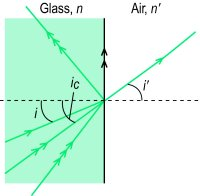Fig. A11 Critical angle ic and total internal reflection (i, angle of incidence; i′, angle of refraction)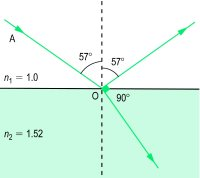Fig. A13 Ray of non-polarized light AO is incident to the surface at the polarizing angle. Most of the light is transmitted across the surface and partially polarized, the rest being reflected and polarized maximally (i.e

 Table A8 Critical angle (in degrees) beyond which all the light is reflected at the surface separating various transparent substances from air or water substance refractive index n critical angle ic in contact with air n′ = 1 in contact with water n′ = 1.333 water 1.333 48.6 - spectacle crown glass 1.523 41.0 61.1 flint glass (dense) 1.62 38.1 55.4 flint glass (extra dense) 1.706 35.9 51.4 PMMA 1.49 42.2 63.5 CR-39 1.498 41.9 62.9 polycarbonate 1.586 39.1 57.2 diamond 2.42 24.4 33.4

 Table A9 Relationship between viewing distance and total convergence in metre angles and prism dioptres for 4 interpupillary distances (PD in cm) object distance from cornea (cm) metre angle (ma) convergence (in prism dioptres) PD: 6.0 6.4 6.8 7.0 200 0.5 3.0 3.2 3.4 3.5 100 1.0 6.0 6.4 6.8 7.0 67 1.5 9.0 9.6 10.2 10.5 50 2.0 12.0 12.8 13.6 14.0 40 2.5 15.0 16.0 17.0 17.5 33 3.0 18.0 19.2 20.4 21.0 25 4.0 24.0 25.6 27.2 28.0 20 5.0 30.0 32.0 34.0 35.0 16 6.25 37.5 40.0 42.5 43.7 14 7.14 42.8 45.7 48.5 50.0

## an·gle

(ang'gĕl) [TA]
Meeting point of two lines or planes; figure formed by the junction of two lines or planes; space bounded on two sides by converging lines or planes.
[L. angulus]

## angle

the space or figure formed by two diverging lines, measured as the number of degrees one would have to be moved to coincide with the other.

cardiodiaphragmatic angle
that formed by the junction of the shadows of the heart and diaphragm in radiographs.
costovertebral angle
the angle formed on either side of the vertebral column between the last rib and the lumbar vertebrae.
filtration angle
the angle between the iris and cornea at the periphery of the anterior chamber of the eye, through which the aqueous humor readily permeates. Called also angle of the iris.
glenoid angle
the angle of the scapula at the glenoid cavity, or ventral end, of the bone. The cranial and caudal angles are at the dorsal border of the scapula.
angle of inclination
angle of jaw
the junction of the ventral and caudal borders of the lower jaw.
nasofrontal angle
see stop.
oral lip angle
angle of union of the oral lips. Called also commissura labiorum.
sole angle
the angle formed by the inflection of the wall of the hoof to form the bars on the sole of the horse's foot. Called also angulus soleae medialis, lateralis.
Site: Follow: Share:
Open / Close